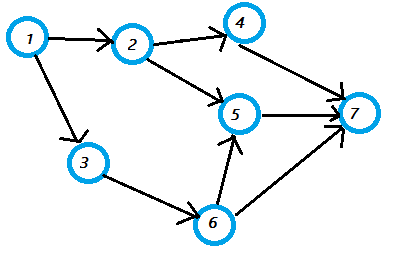# Random Wits

Life is too short for a diary

## Random

\$ random stuff     ├── tags     ├── bookshelf     ├── resources     ├── quotes     ├── habits     └── about me
+ say Hello

Sun 10 Nov 2019

### Getting started with Graph Part 1

Tags: graph java code

Graph are data structures made of vertices & edges. There are lots of interesting problems that can be solved using graphs. Part 1 covers the basic for creating a template for graph data structure.

First we need to create a vertex. A vertex is a fundamental unit of graph. A graph is a set of points (vertices) connected by lines (edges).

## Create a vertex

First we need to create a vertex. A vertex is a fundamental unit of graph. A graph is a set of points (vertices) connected by lines (edges).

class Vertex {

String label;

public Vertex(String label) {
this.label = label;
}
}


## Represent a graph

We need a data structure to represent our graph. We have two choices

So we have a graph like below1 means edge between two vertex where as 0 means no edge

 1 2 3 4 5 6 7 1 0 1 1 0 0 0 0 2 0 0 0 1 1 0 0 3 0 0 0 0 0 1 0 4 0 0 0 0 0 0 1 5 0 0 0 0 0 0 1 6 0 0 0 0 1 0 1 7 0 0 0 0 0 0 0

## Using Adjacency List (we’ll be using this for efficiency)

Here each vertex correspond to a linked list of outgoing edges

 1 → 2, 3 2 → 4, 5 3 → 6 4 → 7 5 → 7 6 → 5 , 7 7
class Vertex {

String label;

public Vertex(String label) {
this.label = label;
}
}

class Graph {
private Map<Vertex, List<Vertex>> adjList = new HashMap<>();
}



## Add lots of vertex (or vertices)

We now need to add add vertex (if it doesn’t exist) to our graph using method addVertex

class Vertex {

String label;

public Vertex(String label) {
this.label = label;
}

return label.equals(this.label);
}
}

class Graph {
private Map<Vertex, List<Vertex>> adjList = new HashMap<>();

for (Map.Entry<Vertex, List<Vertex>> entry : adjList.entrySet()) {
Vertex temp = entry.getKey();

return;
}
}

}
}



We need to add edges between two vertex (if not already existing)


class Vertex {
...
public String getKey() { return this.label; }
}
class Graph {
...
public void addEdge(String label1, String label2) {

Vertex v1 = new Vertex(label1);
Vertex v2 = new Vertex(label2);

for (Map.Entry<Vertex, List<Vertex>> entry : adjList.entrySet()) {
Vertex temp = entry.getKey();
}
}
}

}


## Is that it?

Yup. It’s a basic template for a simple graph. Below is the full code

class Vertex {

String label;

public Vertex(String label) {
this.label = label;
}

return label.equals(this.label);
}

public String getKey() { return this.label; }
}

class Graph {
private Map<Vertex, List<Vertex>> adjList = new HashMap<>();

for (Map.Entry<Vertex, List<Vertex>> entry : adjList.entrySet()) {
Vertex temp = entry.getKey();

return;
}
}

}

public void addEdge(String label1, String label2) {

Vertex v1 = new Vertex(label1);
Vertex v2 = new Vertex(label2);

for (Map.Entry<Vertex, List<Vertex>> entry : adjList.entrySet()) {
Vertex temp = entry.getKey();
}
}
}
}

public class Main {
public static void main(String[] args) {
Graph graph = new Graph();